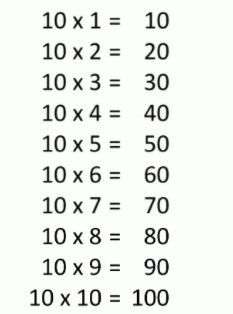# 10 Times Table- Learn Table Of 10 : Multiplication Table Of 10

Safalta expert Published by: Yashaswi More Updated Thu, 26 May 2022 01:59 AM IST

## Highlights

Check out how to learn the 10 Times table easily here at Safalta.com

10 times table is one of the easiest tables to memorize. An easy way to learn the table of 10 is to add a zero after every number you are multiplying with and you have the answer. So, let's learn and understand in detail about the amazing 10 times table in this mini-lesson plan.For , in a classroom there 15 rows and each row consist 10 chairs    .How many total chairs are present ? Answer, the total number chairs will be 15 x 10 = 150 . Join Safalta School Online and prepare for Board Exams under the guidance of our expert faculty. Our online school aims to help students prepare for Board Exams by ensuring that students have conceptual clarity in all the subjects and are able to score their maximum in the exams.

1. 10 times table chart
2. Multiplication table of 10
3. 10 times table
4. tips for 10 times table
5. table of 10 upto 20

## 10 Times Table Chart:## Multiplication Table of 10

Learning the multiplication table of 10 has an advantage while solving mathematical problems and understanding number patterns. Go through the 10 times table that is given below to help you solve math problems quicker.

## Tips for 10 Times Table

• Table of 10 is the easiest to memorize. The digit at the unit's place of the multiples of 10 is always 0.
• Simply write the natural numbers followed by a 0 to obtain the 10 times table.

## What is the importance of learning Table 2 to 20?

For making mathematical section easier, memorising table 2 to 20 is important.

## How can I learn tables easily?

Daily recite the table as mentioned in the article twice and thrice.

• 10 x 1 = 10.
• 10 x 2 = 20.
• 10 x 3 = 30.
• 10 x 4 = 40.
• 10 x 5 = 50.
• 10 x 6 = 60.
• 10 x 7 = 70.
• 10 x 8 = 80.

## How do you describe a 10 times table?

The 10 times table is: 1 × 10 = 10. 2 × 10 = 20. 3 × 10 = 30.
...
1. The 10 times table is made by counting up in tens.
2. All numbers in the ten times table end in a zero.
3. To multiply a whole number by ten, simply put a zero digit on the end of it.
4. This rule makes the ten times table the easiest times table to learn.

## Which is the most easiest table?

10 is the one of the most easiest tables to learn.